Next: 5.4 Fast Diffusivity Paths Up: 5. Simulation Studies of Previous: 5.2.2 The Role of

# 5.3 Stress Effect on Diffusivity

The effect of mechanical stress on the diffusivity and, consequently, on the material transport due to electromigration is analyzed. Consider the copper dual-damascene interconnect structure shown in Figure 5.13. The structure is initially at a temperature of 500C and then cools down to about 100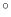C. Upon cooling from such a high temperature, mechanical stress develops in the copper line due to the large difference of the thermal expansion coefficients between the copper and the surrounding materials (Table 5.2). The three stress components,,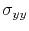, and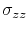along the upper line, via, and lower line are shown in Figure 5.14. Here, the boundary at the bottom of the passivation is assumed to be fixed, simulating the mechanical constraint imposed by a thick substrate.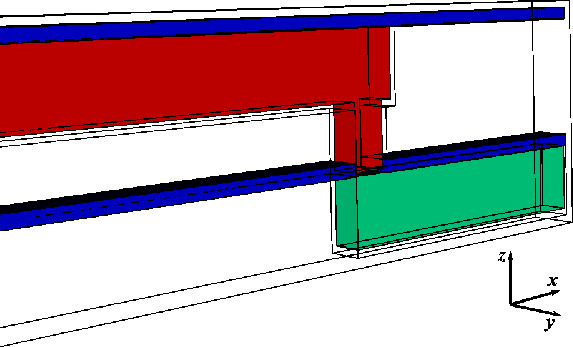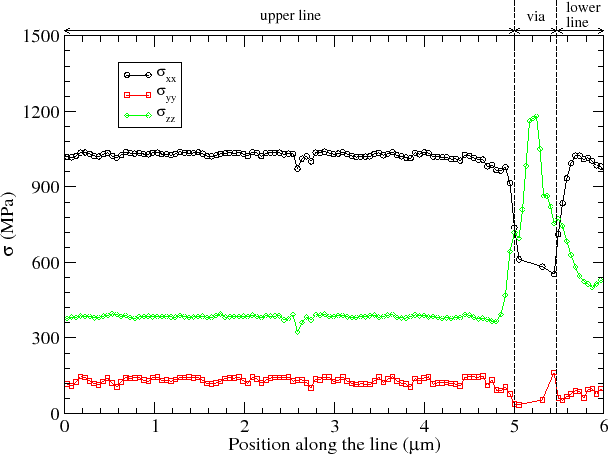One can see that the stress state in the line strip and in the via are rather different. In the line strip, thecomponent has a magnitude of about 1 GPa and is much larger than the other components, whereis about 360 MPa, andabout 120 MPa. The lower stress level foris due to the small dimension of the line in this direction, so that the mechanical constraint imposed by the boundary condition at the bottom of the passivation is small . In the via regionis reduced to 600 MPa, while thecomponent significantly increases, reaching 1.2 GPa. This behavior was already observed by Paik et al.  and Suzuki et al. . A high value ofdevelops due to the small dimensions of the via in the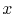anddirections in combination with the constraints imposed in the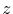direction by the boundary condition at the bottom surface of the passivation, and by the barrier and capping layer, since both have larger elastic modulus than the copper.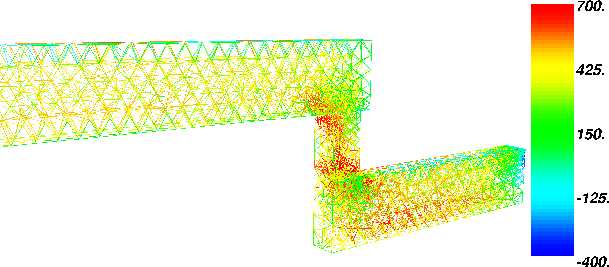(a)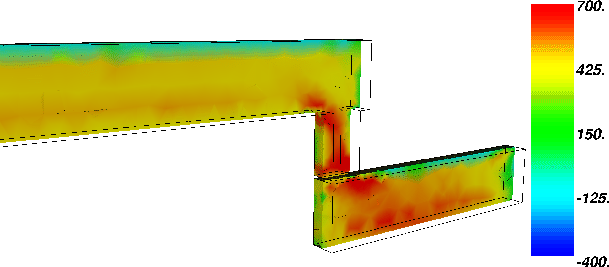(b)

Figure 5.15 shows the hydrostatic stress in the metal lines. The hydrostatic stress distribution has an important influence on the total material transport, as the gradient of hydrostatic stress is an additional driving force for atom migration. The hydrostatic stress is not uniform throughout the copper lines. High stresses develop at the bottom of the via in the top line and at the edge of the via intersecting with the capping layer in the bottom line.

The interconnect is now subjected to an electromigration test at 100C and 2 MA/cm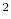, where the current flows from the upper to the lower line. The Ta barrier layer at the bottom of the via acts as a blocking boundary for diffusion, so that the electromigration flux leads to a build-up of vacancies at this site, as shown in Figure 5.16. An interesting observation is that the peak of the vacancy concentration is higher at the outer interfaces than in the bulk of the via. This is a consequence of the non-uniform hydrostatic stress distribution, as vacancies are driven by the high stress gradients present in the via region.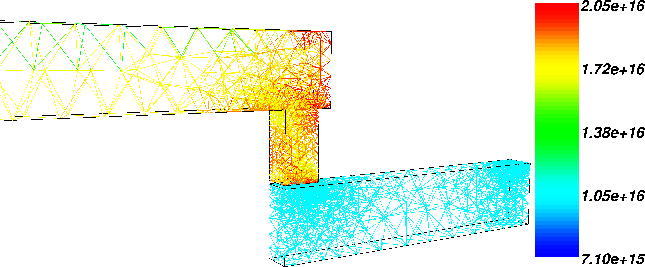In order to show the effect of the stress components (Figure 5.14) on the vacancy transport, three cases are considered. First, it is assumed that the vacancy diffusivity is a constant scalar quantity independent of stress, following the usual Arrhenius law (2.10), with pre-exponential factor and activation energy given in Table 5.3. Then, the diffusivity is treated as a scalar quantity which is affected by the hydrostatic stress according to equation (3.13). In the third case, the effect of the individual stress components is taken into account, yielding a tensorial diffusivity, as derived in Section 3.2.2. The vacancy build-up at the bottom of the via is shown in Figure 5.17. The simulations are carried out at a temperature commonly used in accelerated tests, 300C, and at a temperature close to that found at typical operating conditions, 100C.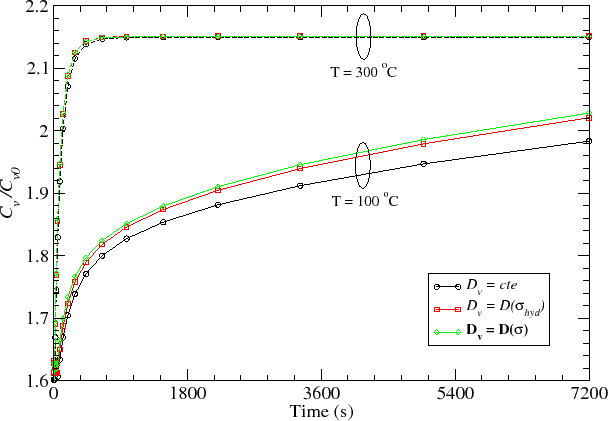One can see that, at the elevated temperature, the vacancy build-up is practically identical for all cases, that is, vacancy transport is not affected by the stress distribution. This means that the diffusivity dependence on stress is weak. Thus, the diffusivity can be considered to be isotropic and can be well described by a scalar quantity. On the other hand, at real operating conditions a clear distinction in vacancy evolution is observed. In this case, the tensile stress originated from the thermal process leads to an increased diffusivity, which, in turn leads to a higher vacancy flux along the conductor lines and a higher vacancy concentration at the via. Thus, the diffusivity dependence on stress becomes an important factor to be considered. The addition of the hydrostatic stress dependence on the scalar diffusivity represents already an improvement in the diffusivity description. Nevertheless, the large difference in magnitudes of the individual components of the stress, as shown in Figure 5.14, certainly leads to an anisotropic diffusion process which can only be described by the tensorial diffusivity, as derived in Section 3.2.2.

The different behavior at the different temperatures is a consequence of the stress magnitude which develops in the structure after the thermal cycle. At an elevated temperature, the stresses which result from the cooling process are smaller, since the difference between the initial temperature and the test temperature is smaller. Therefore, the stress level produced at elevated temperature tests is unlikely to cause a significant variation of the diffusion coefficient. However, it is shown that a different behavior is expected as soon as the test temperature approaches the use condition, where the residual stresses from the fabrication process become large enough to influence the vacancy diffusivity.

These results have an important consequence for the extrapolation of lifetimes obtained from accelerated tests to use conditions. They indicate that the activation energy for the diffusion process obtained from accelerated tests is likely to be wrong, since the stress effect on diffusion has a different impact at the accelerated tests and at the use conditions. This situation can be partially improved by introducing a hydrostatic stress dependence on the diffusion coefficient. However, the anisotropic effect cannot be estimated, since a detailed picture of the stress components cannot be experimentally determined. Thus, the developed model provides a useful insight into this problem.Next: 5.4 Fast Diffusivity Paths Up: 5. Simulation Studies of Previous: 5.2.2 The Role of

R. L. de Orio: Electromigration Modeling and Simulation